# Convert a pipeline with a LightGBM regressor#

The discrepancies observed when using float and TreeEnsemble operator (see Issues when switching to float) explains why the converter for LGBMRegressor may introduce significant discrepancies even when it is used with float tensors.

Library lightgbm is implemented with double. A random forest regressor with multiple trees computes its prediction by adding the prediction of every tree. After being converting into ONNX, this summation becomes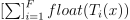, where F is the number of trees in the forest,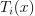the output of tree i and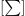a float addition. The discrepancy can be expressed as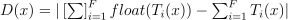. This grows with the number of trees in the forest.

To reduce the impact, an option was added to split the node TreeEnsembleRegressor into multiple ones and to do a summation with double this time. If we assume the node if split into a nodes, the discrepancies then become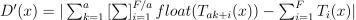.

In 2022, onnx and onnxruntime updated the specifications of TreeEnsemble operators and they can now support double thresholds (see TreeEnsembleRegressor v3). That would be the recommended option to reduce the discrepancies.

## Train a LGBMRegressor#

import warnings
import time
import timeit
from packaging.version import Version
import numpy
from pandas import DataFrame
import matplotlib.pyplot as plt
from tqdm import tqdm
from lightgbm import LGBMRegressor
from onnxruntime import InferenceSession
from skl2onnx import update_registered_converter
from skl2onnx.common.shape_calculator import calculate_linear_regressor_output_shapes  # noqa
from mlprodict.onnx_conv import to_onnx
from onnxmltools import __version__ as oml_version
from onnxmltools.convert.lightgbm.operator_converters.LightGbm import convert_lightgbm  # noqa

N = 1000
X = numpy.random.randn(N, 20)
y = (numpy.random.randn(N) +
numpy.random.randn(N) * 100 * numpy.random.randint(0, 1, 1000))

reg = LGBMRegressor(n_estimators=1000)
reg.fit(X, y)

LGBMRegressor(n_estimators=1000)
In a Jupyter environment, please rerun this cell to show the HTML representation or trust the notebook.

## Register the converter for LGBMRegressor#

The converter is implemented in onnxmltools: onnxmltools…LightGbm.py. and the shape calculator: onnxmltools…Regressor.py.

def skl2onnx_convert_lightgbm(scope, operator, container):
options = scope.get_options(operator.raw_operator)
if 'split' in options:
if Version(oml_version) < Version('1.9.2'):
warnings.warn(
"Option split was released in version 1.9.2 but %s is "
"installed. It will be ignored." % oml_version)
operator.split = options['split']
else:
operator.split = None
convert_lightgbm(scope, operator, container)

update_registered_converter(
LGBMRegressor, 'LightGbmLGBMRegressor',
calculate_linear_regressor_output_shapes,
skl2onnx_convert_lightgbm,
options={'split': None})


## Convert#

We convert the same model following the two scenarios, one single TreeEnsembleRegressor node, or more. split parameter is the number of trees per node TreeEnsembleRegressor.

model_onnx = to_onnx(reg, X[:1].astype(numpy.float32),
target_opset={'': 17, 'ai.onnx.ml': 3})

model_onnx_split = to_onnx(reg, X[:1].astype(numpy.float32),
target_opset={'': 17, 'ai.onnx.ml': 3},
options={'split': 100})


We create another model using the ai.onnx.ml == 3. Node thresholds are stored in doubles and not in floats anymore.

model_onnx_64 = to_onnx(reg, X[:1].astype(numpy.float64),
target_opset={'': 17, 'ai.onnx.ml': 3},
rewrite_ops=True)


## Discrepancies#

sess = InferenceSession(model_onnx.SerializeToString(),
providers=['CPUExecutionProvider'])
sess_split = InferenceSession(model_onnx_split.SerializeToString(),
providers=['CPUExecutionProvider'])

X32 = X.astype(numpy.float32)[:500]
expected = reg.predict(X32)
got = sess.run(None, {'X': X32}).ravel()
got_split = sess_split.run(None, {'X': X32}).ravel()

disp = numpy.abs(got - expected).sum()
disc_split = numpy.abs(got_split - expected).sum()

print(f"sum of discrepancies 1 node: {disp}")
print(f"sum of discrepancies split node: {disc_split}, "
f"ratio: {disp / disc_split}")

sum of discrepancies 1 node: 6.853524745783303e-05
sum of discrepancies split node: 2.0830457483485063e-05, ratio: 3.2901460523452055


The sum of the discrepancies were reduced 4, 5 times. The maximum is much better too.

disc = numpy.abs(got - expected).max()
disc_split = numpy.abs(got_split - expected).max()

print("max discrepancies 1 node", disc)
print("max discrepancies split node", disc_split, "ratio:", disc / disc_split)

max discrepancies 1 node 1.3420434359368016e-06
max discrepancies split node 3.822674381481761e-07 ratio: 3.5107448398903207


Let’s compare with the double thresholds. We compare the inputs into float first and then in double to make sure they are the same.

sess_64 = InferenceSession(model_onnx_64.SerializeToString(),
providers=['CPUExecutionProvider'])

X64 = X32.astype(numpy.float64)
expected_64 = reg.predict(X64)
got_64 = sess_64.run(None, {'X': X64}).ravel()
disc_64 = numpy.abs(got_64 - expected_64).sum()
disc_max64 = numpy.abs(got_64 - expected_64).max()
print(f"sum of discrepancies with doubles: sum={disc_64}, max={disc_max64}")

sum of discrepancies with doubles: sum=9.069053242884979e-06, max=1.1771164043494764e-07


## Processing time#

The processing time is slower but not much.

print("processing time no split",
timeit.timeit(
lambda: sess.run(None, {'X': X32}), number=150))
print("processing time no split with double",
timeit.timeit(
lambda: sess_64.run(None, {'X': X64}), number=150))
print("processing time split",
timeit.timeit(
lambda: sess_split.run(None, {'X': X32}), number=150))

processing time no split 0.773850008030422
processing time no split with double 0.7656510029919446
processing time split 0.8690498230280355


## Split influence#

Let’s see how the sum of the discrepancies moves against the parameter split.

res = []
for i in tqdm(list(range(20, 170, 20)) + [200, 300, 400, 500]):
model_onnx_split = to_onnx(reg, X[:1].astype(numpy.float32),
target_opset={'': 17, 'ai.onnx.ml': 3},
options={'split': i})
times = []
for _ in range(0, 4):
begin = time.perf_counter()
sess_split = InferenceSession(model_onnx_split.SerializeToString(),
providers=['CPUExecutionProvider'])
times.append(time.perf_counter() - begin)
times.sort()
got_split = sess_split.run(None, {'X': X32}).ravel()
disc_split = numpy.abs(got_split - expected).max()
res.append(dict(split=i, max_diff=disc_split, time=sum(times[1:3]) / 2))

df = DataFrame(res).set_index('split')
df["baseline"] = disc
df["baseline_64"] = disc_max64
print(df)

  0%|          | 0/12 [00:00<?, ?it/s]
8%|8         | 1/12 [00:11<02:02, 11.09s/it]
17%|#6        | 2/12 [00:21<01:47, 10.75s/it]
25%|##5       | 3/12 [00:32<01:38, 10.97s/it]
33%|###3      | 4/12 [00:43<01:25, 10.71s/it]
42%|####1     | 5/12 [00:53<01:13, 10.56s/it]
50%|#####     | 6/12 [01:03<01:02, 10.47s/it]
58%|#####8    | 7/12 [01:14<00:53, 10.70s/it]
67%|######6   | 8/12 [01:25<00:42, 10.58s/it]
75%|#######5  | 9/12 [01:35<00:31, 10.47s/it]
83%|########3 | 10/12 [01:45<00:20, 10.40s/it]
92%|#########1| 11/12 [01:55<00:10, 10.34s/it]
100%|##########| 12/12 [02:06<00:00, 10.54s/it]
100%|##########| 12/12 [02:06<00:00, 10.58s/it]
max_diff      time  baseline   baseline_64
split
20     1.499505e-07  0.245719  0.000001  1.177116e-07
40     3.415094e-07  0.253975  0.000001  1.177116e-07
60     2.809303e-07  0.275046  0.000001  1.177116e-07
80     3.464011e-07  0.281518  0.000001  1.177116e-07
100    3.822674e-07  0.287719  0.000001  1.177116e-07
120    3.544410e-07  0.292886  0.000001  1.177116e-07
140    3.822674e-07  0.301454  0.000001  1.177116e-07
160    5.536555e-07  0.305995  0.000001  1.177116e-07
200    6.395834e-07  0.297995  0.000001  1.177116e-07
300    3.822674e-07  0.304245  0.000001  1.177116e-07
400    6.267877e-07  0.301312  0.000001  1.177116e-07
500    7.292194e-07  0.311266  0.000001  1.177116e-07


Graph.

fig, ax = plt.subplots(1, 2, figsize=(10, 4))
df[["max_diff", "baseline", "baseline_64"]].plot(
title="Sum of discrepancies against split\n"
"split = numbers of tree per node",
ax=ax)
df[["time"]].plot(title="Processing time against split\n"
"split = numbers of tree per node",
ax=ax)<AxesSubplot: title={'center': 'Processing time against split\nsplit = numbers of tree per node'}, xlabel='split'>


## Conclusion#

The time curve is too noisy to conclude. More measures should be made. The double sum reduces the discrepancies but increases the processing time. It is a tradeoff. The best option is using double for threshold and summation but it requires the latest definition of TreeEnsemble ai.onnx.ml=3.

# plt.show()


Total running time of the script: ( 2 minutes 46.095 seconds)

Gallery generated by Sphinx-Gallery